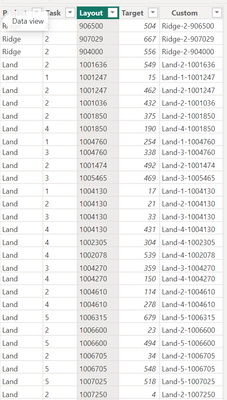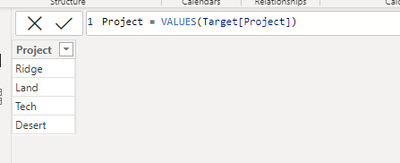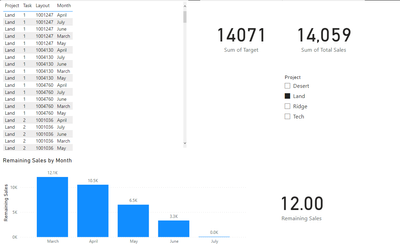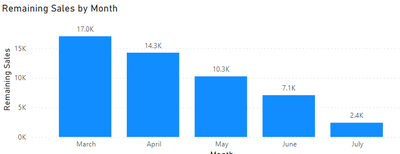cancel
Showing results for
Did you mean:Frequent Visitor

## How to take the target value from one table to subtract from other table?

Hi

I have two tables as follow

Table: TargetTarget Table is unique table. It has four projects, tasks, Layout and target for each.

Table: ActualActual table has month, sales for each project according to Layout and task. At present only three project has been sold.

Both the table has been connected through Custom columnWhat I need?

I need to calculate Remaining sales = Target - Total Sales. Target from Target table and Total Sales from Actual table.

Please note target table doesn't have date or month.

Once I calculate the Remaining sales, I need to visualize by Month.  I did by using below dax measures

``Remaining Sales = SUM(Target[Target]) - SUM(Actual[Total Sales])``The remaining sales values are wrong, I think it's not taking the month properly as target table doesn't have one. Can anyone advise how to fix this?

Attached power bi file. https://we.tl/t-jyuLdOuN0R

1 ACCEPTED SOLUTIONCommunity Support

Hi @Sean500 ,

First create a new table:Then build relationship:Apply the measure:

``````Remaining Sales =
var _a = ALLSELECTED(Project[Project])
return SUM(Target[Target]) - CALCULATE(SUM(Actual[Total Sales]),FILTER(ALL(Actual),[Project] in _a&&[Date]<=MAX('Actual'[Date])))``````

Output:Best Regards,

Jianbo Li

If this post helps, then please consider Accept it as the solution to help the other members find it more quickly.

3 REPLIES 3Frequent Visitor

Thanks @v-jianboli-msft  It is correct against month. However, when I use Project as a filter, the values are not correct Can you please confirm?Community Support

Hi @Sean500 ,

First create a new table:Then build relationship:Apply the measure:

``````Remaining Sales =
var _a = ALLSELECTED(Project[Project])
return SUM(Target[Target]) - CALCULATE(SUM(Actual[Total Sales]),FILTER(ALL(Actual),[Project] in _a&&[Date]<=MAX('Actual'[Date])))``````

Output:Best Regards,

Jianbo Li

If this post helps, then please consider Accept it as the solution to help the other members find it more quickly.Community Support

Hi @Sean500 ,

``Remaining Sales = SUM(Target[Target]) - CALCULATE(SUM(Actual[Total Sales]),FILTER(ALL(Actual),[Date]<=MAX('Actual'[Date])))``

Output:Best Regards,

Jianbo Li

If this post helps, then please consider Accept it as the solution to help the other members find it more quickly.